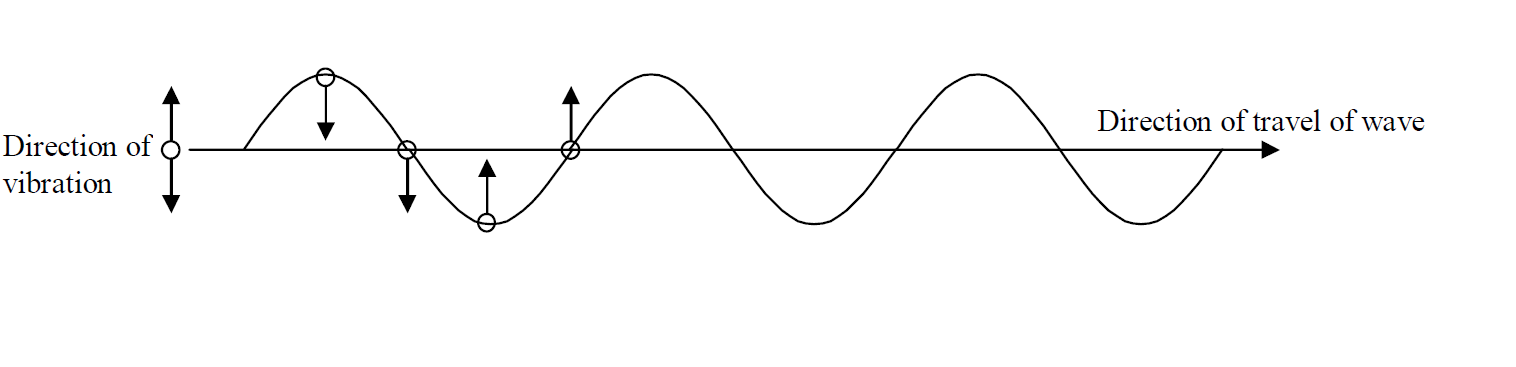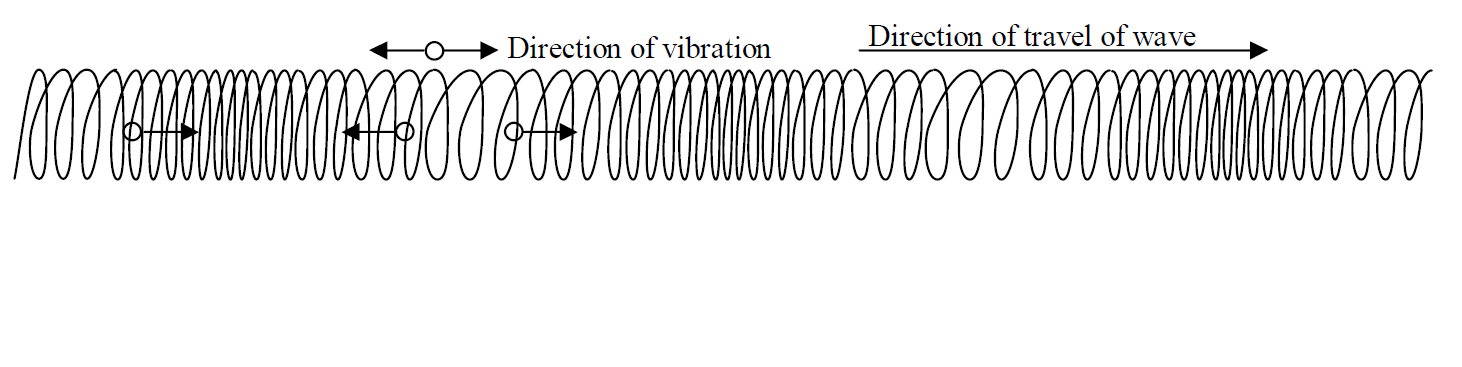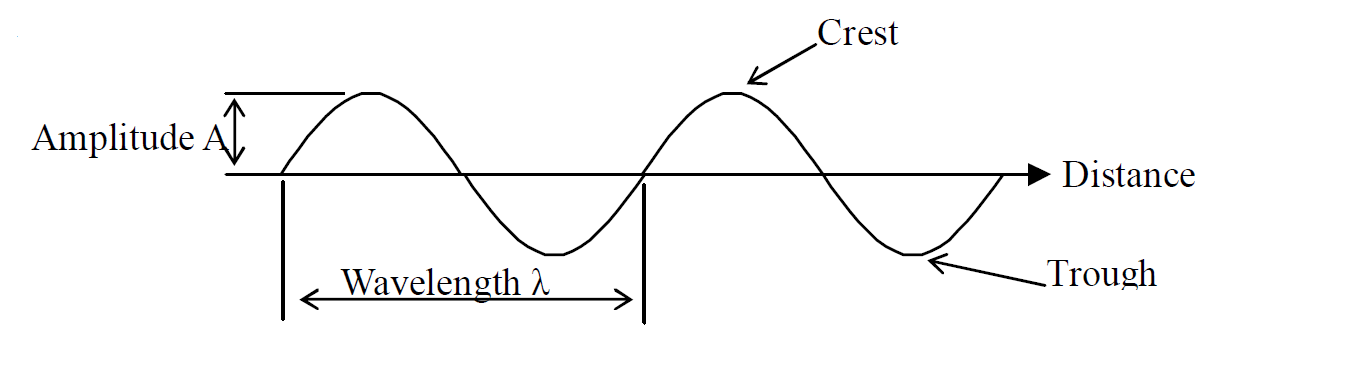# Properties of Waves

A wave is defined as the form that some types of energy take as they move.

e.g. Water wave, Sound wave, Light wave Electromagnetic wave

There are two types of waveforms;

- Transverse wave

- Longitudinal wave

#### Transverse wave

A transverse wave is defined as a wave in which the vibrations of the particles are at right angle to the direction of the travel of the wave.Examples of transverse waves are waves on the rope, light waves, electromagnetic waves

#### Longitudinal wave

A longitudinal wave is defined as a wave in which the vibrations of the particles are parallel to the direction of the travel of the wave.Examples of longitudinal waves are waves on the spring, sound waves

#### Wave terms

##### Amplitude

Amplitude of a wave is the maximum displacement of a particle from its resting position.

- Unit of amplitude is metre [m].

- The symbol is A.

##### Wavelength

Wavelength of a wave is the minimum distance at which the wave repeats itself.

- Unit of wavelength is metre [m].

- The symbol is λ (lambda).Crest: Highest point of a wave .

Trough: Lowest point of a wave

##### Period

Period of a wave is the time taken for one complete vibration of a particle.

- In other words, period means the time it takes to make one wave.

- Unit of period is second [s].

- The symbol is T.

##### Frequency

Frequency of a wave is the number of complete waves produced per second.

- In other words, frequency means the number of waves made in one second.

- Unit of period is Hertz [Hz]. (1Hz = 1s-1)

- The symbol is f.

##### Relationship between frequency and period is;

Formula for frequency

$f=\frac{1}{T}$

f: Frequency [Hz]

T: Period [s]

##### Speed

Speed of a wave is distance traveled by the wave in one second.

- Unit of speed is metre per second [m/s].

- The symbol is v.

Formula for speed of a wave

$v=\mathrm{f\lambda }$

v: Speed of wave [m/s]

f: Frequency [Hz]

λ: Wavelength [m]

#### Example 1

If 100 waves are produced in 5 seconds, what is the frequency?

Solution

Given that

Number of waves = 100

Time taken = 5s

100waves → 5s

x waves → 1

Cross multiply

$x=\frac{\mathrm{100}}{5}$

$x=20Hz$

#### Example 2

A wave source of frequency 1000Hz emits waves of wavelength 0.10m.

(a) What is the period of wave?

(b) What is the speed of wave?

Solutions

(a)

$f=\frac{1}{T}$

Given that

f = 1000Hz

T = ?

$\mathrm{1000}=\frac{1}{T}$

$\mathrm{1000T}=\frac{1}{1}$

$\frac{\mathrm{1000T}}{1000}=\frac{1}{1000}$

Answer: $T=0.001s$

(b)

$v=\mathrm{f\lambda }$

Given that

v = ?

f = 1000HZ

λ = 0.1m

$v=\mathrm{1000}×0.1$

Answer: $v=\mathrm{100m/s}$